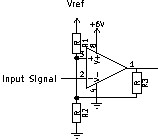# Inverting Op-Amp Schmitt trigger threshold

I'm pretty sure I found an error in a textbook, and I would like your advice before I send it in.It's an inverting Schmitt Trigger, built with an Op-Amp. So the Input Signal is on the Inverting Input, R3 is for hysteresis, R1/R2 Voltage Divider determines the threshold.

The textbook implies that to lower the switching threshold to ground, R1 (which goes to Vref, which is positive) should be omitted - (edit: it's not clear whether to remove R1 or bypass it, it just says to use a Resistor from Noninverting to Ground. If interpreted to remove R1, thereby making it infinite, the textbook would be OK, as pointed out by DarenW.)

However, I think R2, which goes to ground, should be omitted, because then the Noninverting Input would see ground and the Op-Amp would compare the Input Signal to Ground, and if it is higher than Ground, it would turn off (as is desired in an Inverting Schmitt Trigger.)

Am I right with my objection?

• What do you think "omit" means here?
– pipe
Oct 8, 2016 at 5:18
• Bypass - so a wire instead of the resistor. Oct 8, 2016 at 5:36
• By "omit" the textbook means to remove, so R1 becomes infinite. That looks good to me. Oct 8, 2016 at 5:46
• It does not use the word 'omit'. It says to "just use a single resistor from noninverting input to ground". I guess that can be interpreted either way, likely means to remove R1, though. Didn't see that. Guess I'll hold my tongue. Should have quoted the book right away in the question. DarenW, if you post that as an answer, I'll accept it. Thanks! Oct 8, 2016 at 6:28
• English is my second language, by the way, so thanks for pointing out subtleties. Oct 8, 2016 at 6:34

Am I right with my objection? No , and here's why.Consider the three circuits.

(1) Without hysteresis we have a simple potential divider and a single threshold.

(2) Adding R3 to the output means that when the output is high (assume = V) then R3 is effectively in parallel with R1 (R1//R3). This gives the high threshold. When the output is low (assume = 0) then R3 is effectively in parallel with R2 (R3//R2). This gives the low threshold value.

(3) Removing (omitting) R1 still leaves us with a potential divider formed from R3 and R2. When the output is low (assume = 0) this gives us 0 x R2/(R2+R3) which is 0. The high threshold is given by V x R2/(R2+R3).

This is exactly what is implied in the book.

Omitting R2 instead of R1 would mean that the + input could never be pulled down to ground but would either be +V or V x R3/(R1+R3)

Note that the assumption is for an ideal op amp. (i.e. V out switches between rail voltages)